# Changing scientific notation to standard form Scientific Notation

• Slides: 11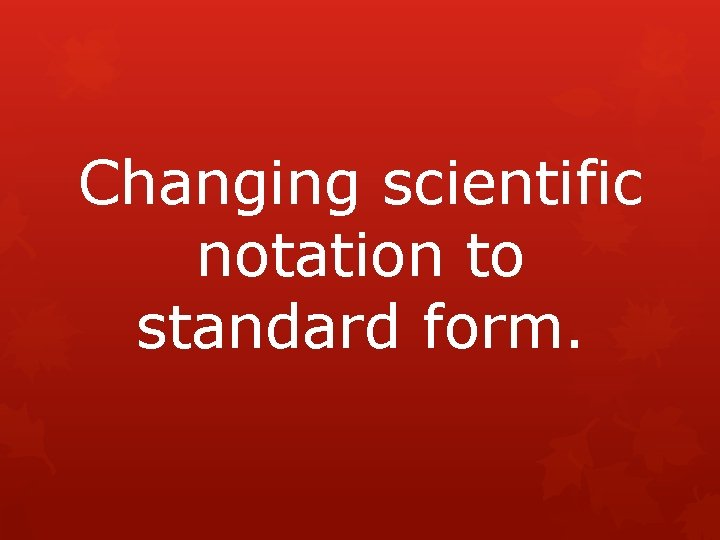Changing scientific notation to standard form.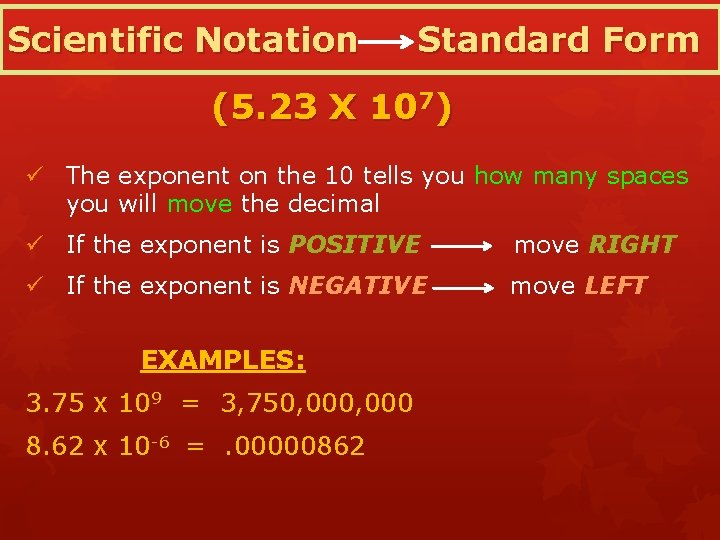Scientific Notation Standard Form (5. 23 X 107) ü The exponent on the 10 tells you how many spaces you will move the decimal ü If the exponent is POSITIVE move RIGHT ü If the exponent is NEGATIVE move LEFT EXAMPLES: 3. 75 x 109 = 3, 750, 000 8. 62 x 10 -6 =. 00000862Example 1: Write the number in standard notation: 1. 35 105 1. 35 100, 000 135, 000 BECAUSE 105 = 100, 000 Think: Move the decimal right places. 5 Helpful Hint A positive exponent means move the decimal to the right, and a negative exponent means move the decimal to the left.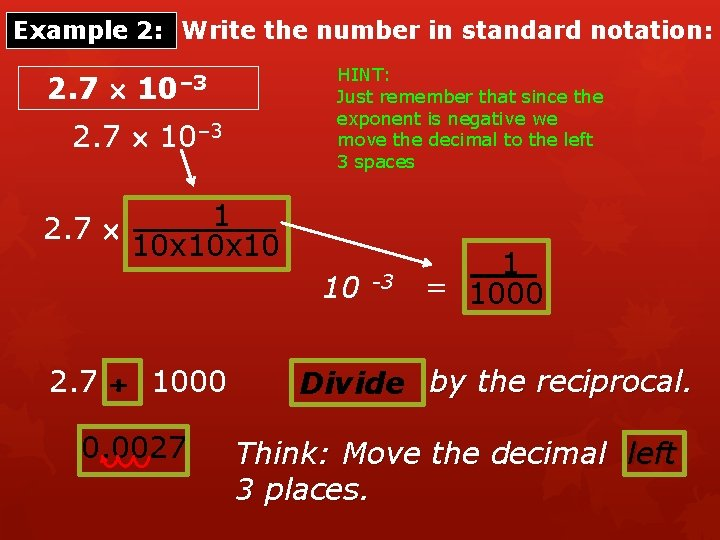Example 2: Write the number in standard notation: 2. 7 HINT: Just remember that since the exponent is negative we move the decimal to the left 3 spaces 10– 3 2. 7 1 10 x 10 10 2. 7 1000 0. 0027 -3 1 = 1000 Divide by the reciprocal. Think: Move the decimal left 3 places.Example 3: Write the number in standard notation: -2. 01 104 HINT: It doesn’t matter that the number (-2. 01) is negative – only the exponent changes things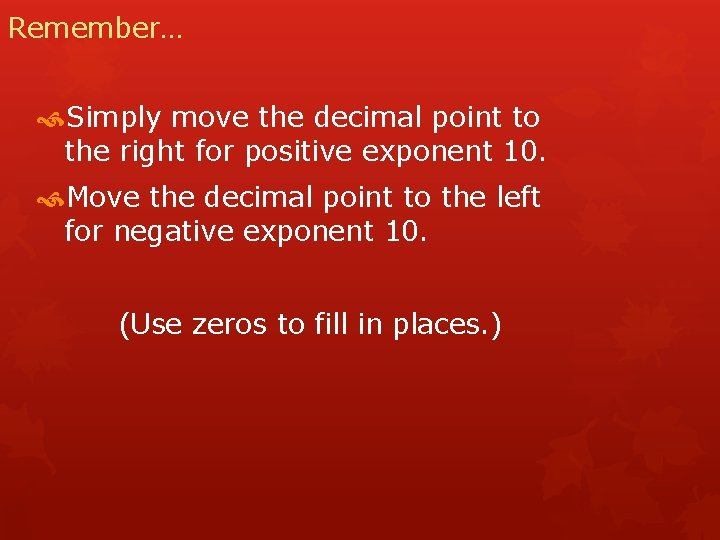Remember… Simply move the decimal point to the right for positive exponent 10. Move the decimal point to the left for negative exponent 10. (Use zeros to fill in places. )Example 4 Given: 5. 093 x 106 Answer: 5, 093, 000 (moved 6 places to the right)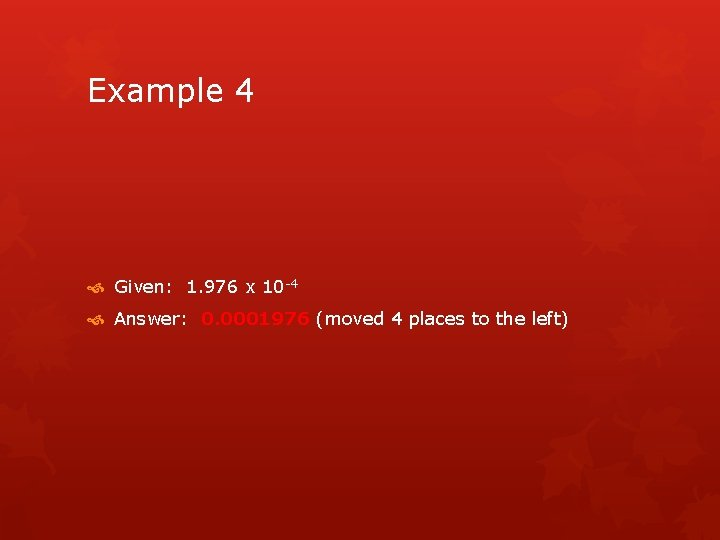Example 4 Given: 1. 976 x 10 -4 Answer: 0. 0001976 (moved 4 places to the left)Ticket Out The Door Write each number in standard notation. 1. 1. 72 2. 6. 9 10– 3 104 17, 200 0. 0069 Write each number in scientific notation. 3. 0. 0053 5. 3 10– 3 4. 57, 000 5. 7 107 5. Order the numbers from least to greatest: 2 10– 4 , 9 10– 5 , 7 10– 5 7 10– 5, 9 10– 5, 2 10– 4Even More Practice Below is a list of links to games and activities all having to do with scientific notation. http: //www. aaamath. com/dec 71 i-dec 2 sci. html http: //janus. astro. umd. edu/cgi-bin/astro/scinote. pl http: //www. sciencejoywagon. com/physicszone/lesson/00 ge nral/dectosci. htm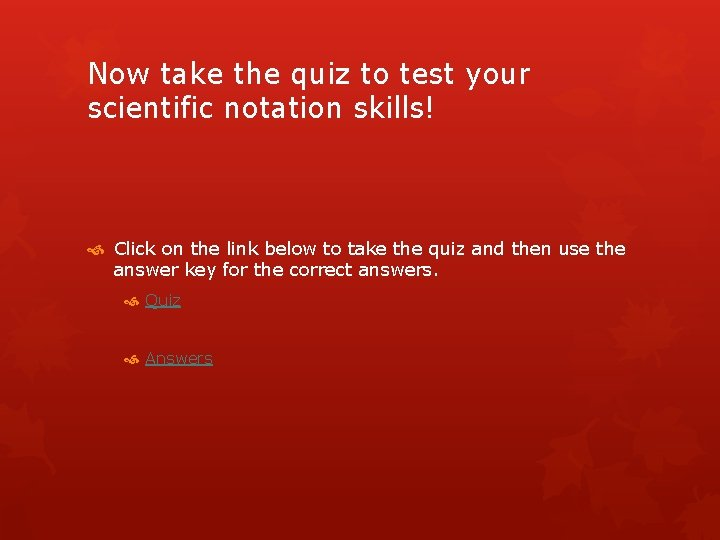Now take the quiz to test your scientific notation skills! Click on the link below to take the quiz and then use the answer key for the correct answers. Quiz Answers